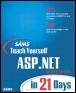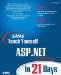# Useful VB.NET Functions

 IOTA^_^Sams Teach Yourself ASP.NET in 21 Days, Second EditionBy Chris Payne Table of Contents Day 3.  Using Visual Basic.NET and C#

If you are familiar with prior versions of VB.NET, you may wonder where all of your favorite functions and subroutines have gone. Many have been incorporated in some way or another into the classes of the .NET Framework. Many, however, are still part of VB.NET. This section briefly describes many common and useful VB.NET functions that you'll be using throughout the book. This section is by no means a complete reference, but it will provide some general information.

(If you're wondering where these functions are in C#, you'll find most of them built into the .NET Framework classes. You'll see their usage as we progress through this book.)

Tables 3.5, 3.6, and 3.7 introduce many of these common functions.

##### Table 3.5. Date/Time Functions
Function Description
dateDiff(dateinterval, date1, date2[, firstdayofweek [, firstdayofyear]]) Returns a number specifying the number of dateintervals between date1 and date2. dateinterval can be yyyy (year), q (quarter), m (month), y (day of year), d (day), w (weekday), ww (week), h (hour), n (minute), s (second).
day(datetime) Returns an integer from 1 to 31 specifying the day of the month.
dayofweek Returns an integer specifying the day of the week (0 = Sunday, 6 = Saturday); this is a property of the datetime data type.
hour(time) Returns an integer from 0 to 23 specifying the hour of the day.
isdate(datetime) Returns a Boolean specifying if the supplied datetime is recognized as a valid date.
minute(time) Returns an integer from 0 to 59 specifying the minute of the hour.
month(datetime) Returns an integer from 1 to 12 specifying the month.
now() Returns a datetime data type specifying the current date and time according to your computer.
second(time) Returns an integer from 0 to 59 specifying the second of the minute.
year(datetime) Returns an integer representing the year (from 1 to 9999).

##### Table 3.6. Math Functions
Function Description
abs(value) The absolute value of value
atan(value) The arctangent of value
cos(value) Cosine of value
exp(value) e^value
fix(value) Returns the integer portion of a number, rounding up for negative numbers
hex(value) Changes base 10 to hexadecimal
int(value) Returns the integer portion of a number, rounding down for negative numbers
log(value) Returns the natural logarithm
oct(value) Changes base 10 to octal
rnd Returns a random number
round(value [, dec]) Rounds to integer, or with dec decimal places
sin(value) Sine of value
sqrt(value) Square root of value
tan(value) Tangent of value

##### Table 3.7. String Functions
Function Description
instr([start, ]string1, string2[, compare]) Returns a number specifying the first position of string2 in string1, or 0 otherwise; compare can be 0 (BinaryCompare) or 1 (TextCompare)
left(string, length) Returns a string containing a specified number of characters from the left side of a string
len(string | variable) Returns a number containing the length of a string or number of bytes required to store a variable
mid(string, start[, length])

Returns a string containing a specified number of characters from another string:

` Dim strstring as string = mid("hello", 3) `

This returns "llo"

replace(expression, find, replace[, start[, count [, compare]]]) Replaces find in the expression with replace, starting at start; count is number of replacements to perform; default is 1 (all possible replacements); compare is similar to instr
right(string, length) Returns a string containing a specified number of characters from the right side of a string

 IOTA^_^
 TopSams Teach Yourself ASP.NET in 21 Days (2nd Edition)
ISBN: 0672324458
EAN: 2147483647
Year: 2003
Pages: 307
Authors: Chris Payne

Similar book on Amazon